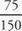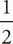# SAT Math Multiple Choice Question 159: Answer and Explanation

### Test Information

Question: 159

9. Clark's Rule is a formula used to determine the correct dosage of adult over-the-counter medicine a child can receive. The child's weight, in pounds, is divided by 150, and the result is multiplied by the adult dose of the medicine. A mother needs to give her daughter acetaminophen, which has an adult dose of 1,000 milligrams. She does not know her daughter's exact weight, but she knows the weight is between 75 and 90 pounds. Which of the following gives the range of correct dosage, d, in milligrams of acetaminophen the daughter could receive?

• A. 50 < d < 60
• B. 500 < d < 600
• C. 1,000 < d < 1,200
• D. 1,600 < d < 2,000

B Start by calculating the least amount of acetaminophen the child needs. If the child is 75 pounds, then the amount of acetaminophen needed can be calculated as× 1,000 =× 1,000 = 500. Since, only (B) gives 500 as the low-end value, the correct answer is (B).